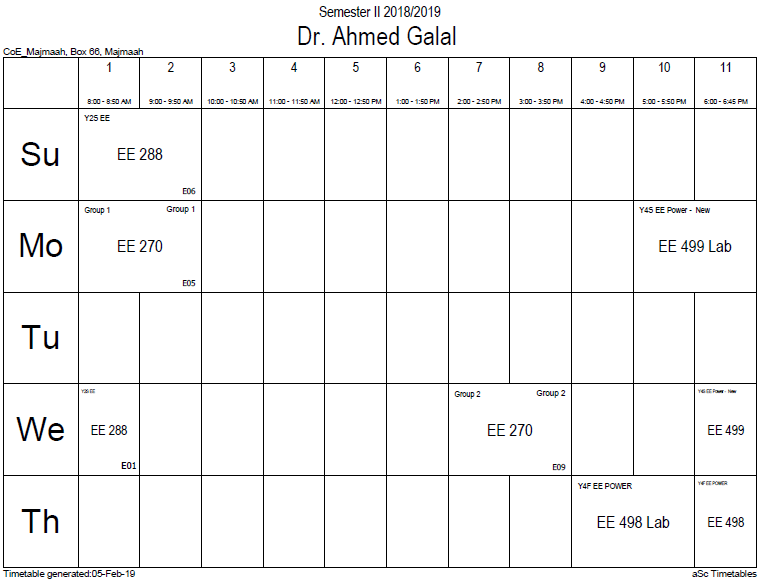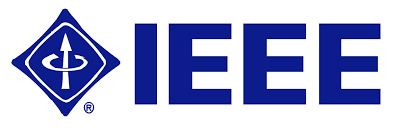Linear motion

Linear motion (also called rectilinear motion) is motion along a straight line, and can therefore be described mathematically using only one spatial dimension. The linear motion can be of two types: uniform linear motion with constant velocity or zero acceleration; non uniform linear motion with variable velocity or non-zero acceleration. The motion of a particle (a point-like object) along a line can be described by its position$x$, which varies with$t$ (time). An example of linear motion is an athletic running 100m along a straight track.

Linear motion is the most basic of all motion. According to Newton's first law of motion, objects that experience no net force will continue to move in a straight line with a constant velocity until they are subject to a net force. Under everyday circumstances, external forces such as gravity and friction can cause an object to change the direction of its motion, so that its motion cannot be described as linear.

One may compare linear motion to general motion. In general motion, a particle's position and velocity are described by vectors, which have a magnitude and direction. In linear motion, the directions of all the vectors describing the system are equal and constant which means the objects move along the same axis and do not change direction. The analysis of such systems may therefore be simplified by neglecting the direction components of the vectors involved and dealing only with the magnitude.

Neglecting the rotation and other motions of the Earth, an example of linear motion is that of a ball thrown straight up and falling back straight down.

Office HoursMonday 10 -2

Tuesday 10-12

Thursday 11-1

My TimetableContactsemail: [email protected]

Phone: 2570

Welcome

Welcome To Faculty of EngineeringIEEEhttp://www.ieee.org/

/

Bookmarkshttp://www.utk.edu/research/

http://science.doe.gov/grants/index.asp

http://www1.eere.energy.gov/vehiclesandfuels/

http://www.eere.energy.gov/

Upcoming ConferencesEngineering quotesTravel Web Siteshttp://www.hotels.com/

http://www.orbitz.com/

http://www.hotwire.com/us/index.jsp

http://www.kayak.com/

Blackboardستقام اختبارات الميدتيرم يوم الثلاثاء 26-6-1440

حسب الجدول المعلن بلوحات الاعلان

Summer trainingThe registration for summer training will start from 5th week of second semesterClass registration week 1

برنامج التجسيرإحصائية الموقع

عدد الصفحات: 2879

البحوث والمحاضرات: 1280

الزيارات: 63988